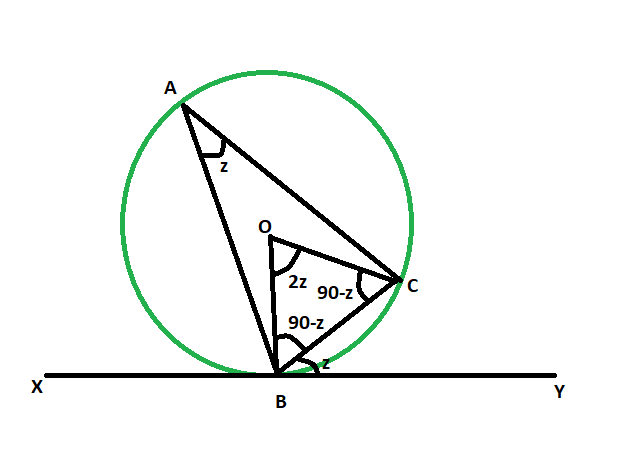Open in App
Not now

# Angle between a chord and a tangent when angle in the alternate segment is given

• Last Updated : 30 May, 2022

Given a circle whose chord and tangent meet at a particular point. The angle in the alternate segment is given. The task here is to find the angle between the chord and the tangent.
Examples:

```Input: z = 48
Output: 48 degrees

Input: z = 64
Output: 64 degrees```Approach

• Let, angle BAC is the given angle in the alternate segment.
• let, the angle between the chord and circle = angle CBY = z
• as line drawn from center on the tangent is perpendicular,
• so, angle OBC = 90-z
• as, OB = OC = radius of the circle
• so, angle OCB = 90-z
• now, in triangle OBC
angle OBC + angle OCB + angle BOC = 180
angle BOC = 180 – (90-z) – (90-z)
angle BOC = 2z
• as angle at the circumference of a circle is half the angle at the centre subtended by the same arc,
so, angle BAC = z
• hence, angle BAC = angle CBYBelow is the implementation of the above approach:

## C++

 `// C++ program to find the angle` `// between a chord and a tangent` `// when angle in the alternate segment is given`   `#include ` `using` `namespace` `std;`   `void` `anglechordtang(``int` `z)` `{` `    ``cout << ``"The angle between tangent"` `         ``<< ``" and the chord is "` `         ``<< z << ``" degrees"` `<< endl;` `}`   `// Driver code` `int` `main()` `{` `    ``int` `z = 48;` `    ``anglechordtang(z);` `    ``return` `0;` `}`

## Java

 `// Java program to find the angle` `// between a chord and a tangent` `// when angle in the alternate segment is given` `import` `java.io.*;`   `class` `GFG ` `{`   `    ``static` `void` `anglechordtang(``int` `z)` `    ``{` `        ``System.out.print( ``"The angle between tangent"` `            ``+ ``" and the chord is "` `            ``+ z + ``" degrees"``);` `    ``}` `    `  `    ``// Driver code` `    ``public` `static` `void` `main (String[] args) ` `    ``{` `        ``int` `z = ``48``;` `        ``anglechordtang(z);` `    ``}` `}`   `// This code is contributed by anuj_67..`

## Python3

 `# Python3 program to find the angle` `# between a chord and a tangent` `# when angle in the alternate segment is given` `def` `anglechordtang(z):`   `    ``print``(``"The angle between tangent"``, ` `          ``"and the chord is"``, z , ``"degrees"``);`   `# Driver code` `z ``=` `48``;` `anglechordtang(z);`   `# This code is contributed` `# by Princi Singh`

## C#

 `// C# program to find the angle` `// between a chord and a tangent` `// when angle in the alternate segment is given` `using` `System;`   `class` `GFG ` `{`   `    ``static` `void` `anglechordtang(``int` `z)` `    ``{` `        ``Console.WriteLine( ``"The angle between tangent"` `            ``+ ``" and the chord is "` `            ``+ z + ``" degrees"``);` `    ``}` `    `  `    ``// Driver code` `    ``public` `static` `void` `Main () ` `    ``{` `        ``int` `z = 48;` `        ``anglechordtang(z);` `    ``}` `}`   `// This code is contributed by anuj_67..`

## Javascript

 ``

Output:

`The angle between tangent and the chord is 48 degrees`

Time Complexity: O(1)

Auxiliary Space: O(1)

My Personal Notes arrow_drop_up
Related Articles